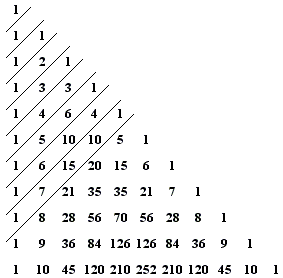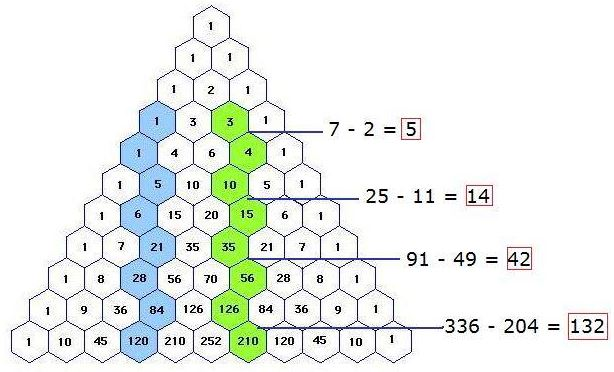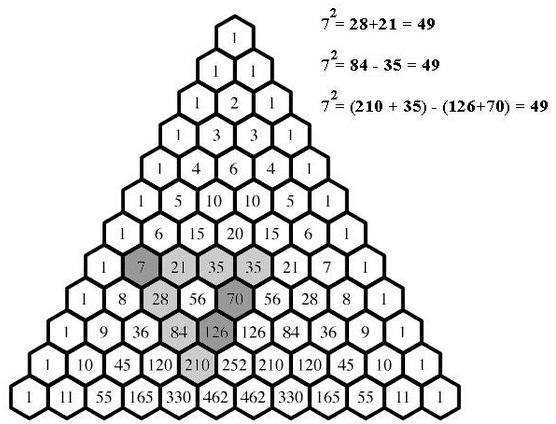# Patterns in Pascal's Triangle

Pascal's Triangle conceals a huge number of various patterns, many discovered by Pascal himself and even known before his time.#### Pascal's Triangle is symmetric

In terms of the binomial coefficients, $C^{n}_{m} = C^{n}_{n-m}.$ This follows from the formula for the binomial coefficient

$\displaystyle C^{n}_{m}=\frac{n!}{m!(n-m)!}.$

It is also implied by the construction of the triangle, i.e., by the interpretation of the entries as the number of ways to get from the top to a given spot in the triangle.

Some authors even considered a symmetric notation (in analogy with trinomial coefficients)

$\displaystyle C^{n}_{m}={n \choose m\space\space s}$

where $s = n - m.$

#### The sum of entries in row $n$ equals $2^{n}$

This is Pascal's Corollary 8 and can be proved by induction. The main point in the argument is that each entry in row $n,$ say $C^{n}_{k}$ is added to two entries below: once to form $C^{n + 1}_{k}$ and once to form $C^{n + 1}_{k+1}$ which follows from Pascal's Identity:

$C^{n + 1}_{k} = C^{n}_{k - 1} + C^{n}_{k},$
$C^{n + 1}_{k+1} = C^{n}_{k} + C^{n}_{k+1}.$

For this reason, the sum of entries in row $n + 1$ is twice the sum of entries in row $n.$ (This is Pascal's Corollary 7.)

As a consequence, we have Pascal's Corollary 9: In every arithmetical triangle each base exceeds by unity the sum of all the preceding bases. In other words, $2^{n} - 1 = 2^{n-1} + 2^{n-2} + ... + 1.$

#### There are well known sequences of numbers

Some of those sequences are better observed when the numbers are arranged in Pascal's form where because of the symmetry, the rows and columns are interchangeable.The first row contains only $1$s: $1, 1, 1, 1, \ldots$
The second row consists of all counting numbers: $1, 2, 3, 4, \ldots$
The third row consists of the triangular numbers: $1, 3, 6, 10, \ldots$
The fourth row consists of tetrahedral numbers: $1, 4, 10, 20, 35, \ldots$
The fifth row contains the pentatope numbers: $1, 5, 15, 35, 70, \ldots$

"Pentatope" is a recent term. Regarding the fifth row, Pascal wrote that ... since there are no fixed names for them, they might be called triangulo-triangular numbers. Pentatope numbers exists in the $4D$ space and describe the number of vertices in a configuration of $3D$ tetrahedrons joined at the faces.

In the standard configuration, the numbers $C^{2n}_{n}$ belong to the axis of symmetry. Numbers $\frac{1}{n+1}C^{2n}_{n}$ are known as Catalan numbers.

Every two successive triangular numbers add up to a square: $(n - 1)n/2 + n(n + 1)/2 = n^{2}.$

#### Hockey Stick PatternIn Pascal's words (and with a reference to his arrangement), In every arithmetical triangle each cell is equal to the sum of all the cells of the preceding row from its column to the first, inclusive (Corollary 2). In modern terms,

(1)

$C^{n + 1}_{m} = C^{n}_{m} + C^{n - 1}_{m - 1} + \ldots + C^{n - m}_{0}.$

Note that on the right, the two indices in every binomial coefficient remain the same distance apart: $n - m = (n - 1) - (m - 1) = \ldots$ This allows rewriting (1) in a little different form:

(1')

$C^{m + r + 1}_{m} = C^{m + r}_{m} + C^{m + r - 1}_{m - 1} + \ldots + C^{r}_{0}.$

The latter form is amenable to easy induction in $m.$ For $m = 0,$ $C^{r + 1}_{0} = 1 = C^{r}_{0},$ the only term on the right. Assuming (1') holds for $m = k,$ let $m = k + 1:$

\begin{align} C^{k + r + 2}_{k + 1} &= C^{k + r + 1}_{k + 1} + C^{k + r + 1}_{k}\\ &= C^{k + r + 1}_{k + 1} + C^{k + r}_{k} + C^{k + r - 1}_{k - 1} + \ldots + C^{r}_{0}. \end{align}

Naturally, a similar identity holds after swapping the "rows" and "columns" in Pascal's arrangement: In every arithmetical triangle each cell is equal to the sum of all the cells of the preceding column from its row to the first, inclusive (Corollary 3).

(2)

$C^{n + 1}_{m + 1} = C^{n}_{m} + C^{n - 1}_{m} + \ldots + C^{0}_{m},$

where the second index is fixed.

#### Parallelogram Pattern(3)

$C^{n + 1}_{m} - 1 = \sum C^{k}_{j},$

where $k \lt n,$ $j \lt m.$ In Pascal's words: In every arithmetic triangle, each cell diminished by unity is equal to the sum of all those which are included between its perpendicular rank and its parallel rank, exclusively (Corollary 4). This is shown by repeatedly unfolding the first term in (1).

#### Fibonacci Numbers

If we arrange the triangle differently, it becomes easier to detect the Fibonacci sequence:The successive Fibonacci numbers are the sums of the entries on sw-ne diagonals:

\begin{align} 1 &= 1\\ 1 &= 1\\ 2 &= 1 + 1\\ 3 &= 1 + 2\\ 5 &= 1 + 3 + 1\\ 8 &= 1 + 4 + 3\\ 13 &= 1 + 5 + 6 + 1 \end{align}

#### The Star of David

The following two identities between binomial coefficients are known as "The Star of David Theorems":

$C^{n-1}_{k-1}\cdot C^{n}_{k+1}\cdot C^{n+1}_{k} = C^{n-1}_{k}\cdot C^{n}_{k-1}\cdot C^{n+1}_{k+1}$ and
$\mbox{gcd}(C^{n-1}_{k-1},\,C^{n}_{k+1},\,C^{n+1}_{k}) = \mbox{gcd}(C^{n-1}_{k},\,C^{n}_{k-1},\, C^{n+1}_{k+1}).$

The reason for the moniker becomes transparent on observing the configuration of the coefficients in the Pascal Triangle.Tony Foster observed that with $k=1,$

$\displaystyle C^{n-2}_{k-1}\cdot C^{n-1}_{k+1}\cdot C^{n}_{k}=\frac{(n-2)(n-1)n}{2}=C^{n-2}_{k}\cdot C^{n-1}_{k-1}\cdot C^{n}_{k+1}$

so that

\displaystyle\begin{align} \prod_{m=1}^{N}\bigg[C^{3m-1}_{0}\cdot C^{3m}_{2}\cdot C^{3m+1}_{1} + C^{3m-1}_{1}\cdot C^{3m}_{0}\cdot C^{3m+1}_{2}\bigg] &= \prod_{m=1}^{N}(3m-2)(3m-1)(3m)\\ &= \prod_{m=1}^{3N}m = (3N)! \end{align}

#### Not without $e$

Harlan Brothers has recently discovered the fundamental constant $e$ hidden in the Pascal Triangle; this by taking products - instead of sums - of all elements in a row:$S_{n}$ is the product of the terms in the $n$th row, then, as $n$ tends to infinity,

$\displaystyle\lim_{n\rightarrow\infty}\frac{s_{n-1}s_{n+1}}{s_{n}^{2}} = e.$

I placed the derivation into a separate file.

#### Catalan Numbers

Tony Foster's post at the CutTheKnotMath facebook page pointed the pattern that conceals the Catalan numbers:I placed an elucidation into a separate file.

#### Sums of the Binomial Reciprocals

A post at the CutTheKnotMath facebook page by Daniel Hardisky brought to my attention to the following pattern:I placed a derivation into a separate file.

#### Squares

As I mentioned earlier, the sum of two consecutive triangualr numbers is a square: $(n - 1)n/2 + n(n + 1)/2 = n^{2}.$ Tony Foster brought up sightings of a whole family of identities that lead up to a square.For example,

$C^{n+2}_{3} - C^{n}_{3} = n^{2}.$

and also

$C^{n+3}_{4} - C^{n+2}_{4} - C^{n+1}_{4} + C^{n}_{4} = n^{2}.$

I placed a derivation into a separate file.

#### Squares of the Binomials

$\displaystyle\sum_{k=0}^{n}(C^{n}_{k})^{2}=C^{2n}_{n}.$

I placed a derivation into a separate file.

#### Cubes

Underfatigble Tony Foster found cubes in Pascal's triangle in a pattern that he rightfully refers to as the Star of David - another appearance of that simile in Pascal's triangle.:

$\displaystyle n^{3}=\bigg[C^{n+1}_{2}\cdot C^{n-1}_{1}\cdot C^{n}_{0}\bigg] + \bigg[C^{n+1}_{1}\cdot C^{n}_{2}\cdot C^{n-1}_{0}\bigg] + C^{n}_{1}.$

Here's his original graphics that explains the designation:There is a second pattern - the "Wagon Wheel" - that reveals the square numbers.

I placed a derivation into a separate file.

### $pi$ in Pascal

This is due to Daniel Hardisky

$\displaystyle\pi = 3+\frac{2}{3}\bigg(\frac{1}{C^{4}_{3}}-\frac{1}{C^{6}_{3}}+\frac{1}{C^{8}_{3}}-\cdot\bigg).$I placed a derivation into a separate file.

### Products of Binomial Coefficients

For integer $n\gt 1,\;$ let $\displaystyle P(n)=\prod_{k=0}^{n}{n\choose k}\;$ be the product of all the binomial coefficients in the $n\text{-th}\;$ row of the Pascal's triangle. Then

$\displaystyle\frac(n+1)!P(n+1)}{P(n)}=(n+1)^{n+1}$

To illustrate,I placed a derivation into a separate file.

Eventually, Tony Foster found an extension to other integer powers:I placed a derivation into a separate file.

### References

1. J. H. Conway and R. K. Guy, The Book of Numbers, Springer-Verlag, NY, 1996.
2. H. Eves, Great Moments in Mathematics After 1650, MAA, 1983
3. Great Books of the Western World, v 33, Encyclopaedia Britannica, Inc., 1952.
4. P. Hilton, D. Holton, J. Pederson, Mathematical Reflections, Springer Verlag, 1997
5. A. R. Kanga, Number Mosaics, World Scientific Co., 1995.
6. R. Graham, D. Knuth, O. Patashnik, Concrete Mathematics, 2nd edition, Addison-Wesley, 1994.
7. J. A. Paulos, Beyond Numeracy, Vintage Books, 1992
8. H.-O. Peitgen et al, Chaos and Fractals: New Frontiers of Science , Springer, 2nd edition, 2004
9. D. E. Smith, History of Mathematics, Dover, 1968
10. D. E. Smith, A Source Book in Mathematics, Dover, 1959
11. D. Wells, The Penguin Dictionary of Curious and Interesting Numbers, Penguin Books, 1987### Pascal's Triangle and the Binomial Coefficients